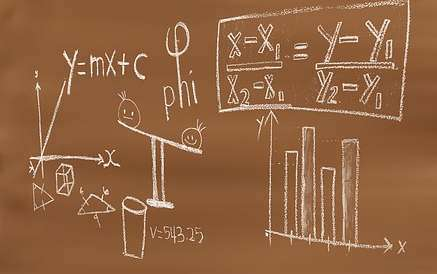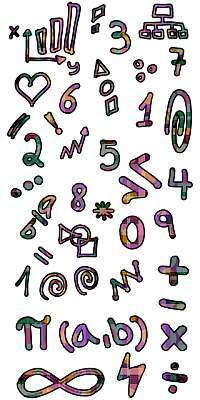# Order of Operations Calculator

Instructions: Use this order of operations calculator to compute an expression following the PEMDAS rules of priority of operations. Please type in a numeric or symbolic expression you want to calculate and simplify in the form box below.Enter a numeric or symbolic expression you want to calculate (Ex: 3/4 + 4/5 *(x-3/4), etc.)

Use this calculator to expand and simplify any valid numeric or symbolic expression that you provide. A valid numeric expression is something like (1/3+1/4)(1/5+1/7), and a valid symbolic expression would be something like (x+3/4)^2 - (x-1/2)^3.

When you have your expression already added in the corresponding box, all you need to do is to click on the "Calculate" button to get all the steps shown. Some simple expressions will require only a few steps to be simplified, but depending on how complicated the original expression is, it could be very labor intensive to fully simplify it.

The idea is to follow the PEMDAS steps, and the golden rule is to always start with internal parentheses, expanding from inside out, following the order of operations specifications.## How to order of operations with fractions?

That is one of the interesting things about PEMDAS: the procedure does not change at all for different operands. Indeed, PEMDAS does not really care about what type of operands you have, it just cares about the order of operations.

Your operands could be numbers or fractions, or even squared roots, and it won't change bit the order that PEMDAS follows.

## What is the correct order of operations for a calculation?

You need to follow this order of operations:

• Step 1: P = Parentheses
• Step 2: E = Exponents
• Step 3: M = Multiplications
• Step 4: D = Divisions
• Step 5: A = Additions
• Step 6: S = Subtractions Multiplications

Notice that this is NOT saying that you will do, for example, ALl multiplications before ALL additions. Indeed, consider the following expression:

$3\times (3+5)$

Which operation would you do first? A misinterpretation of the order of operations rule would be to say "multiplications before additions". In this case we need to focus on the parentheses first, which contains an addition, and we need to simplify the addition inside of the parentheses first. So we do

$3\times (3+5) = 3\times 8 = 24$

So in this case we had to do an addition first, because it order to respect PEMDAS criteria, we needed to deal with the parentheses first.

Normally, a well written expression will not have any ambiguity that needs to be solved with PEMDAS, and typically, it will contain parentheses which will explicitly indicate which operations go first.

It is usually the case that we need to be using the order of operations rules to untie a potential ambiguity that was not dealt with using parentheses.

## How important is it to use the correct order of operation?

It is crucial! It cannot be understated. Without a clear set of rules to address potential ambiguities we could potentially arrive to different answers when starting with the same expression.

You may not think too much about PEMDAS and the order of operation, but it is because you mostly have it internalized, and that usually expressions may come with proper parentheses that eliminate ambiguities.### Example: Order of operation examples

Simplify the following: $$\displaystyle \frac{1}{4}x + \left( \frac{5}{4}x - \frac{5}{6}x\right)$$

Solution: We need to simplify the following expression: $$\displaystyle \frac{1}{4}x + \left( \frac{5}{4}x - \frac{5}{6}x\right)$$.

The following calculation is obtained:

$$\displaystyle \frac{1}{4}x+\frac{5}{4}x-\frac{5}{6}x$$
Grouping the terms with $$x$$
$$= \,\,$$
$$\displaystyle \frac{1}{4}x+\left(\frac{5}{4}-\frac{5}{6}\right)x$$
Simplifying the terms that were grouped with $$x$$
$$= \,\,$$
$$\displaystyle \frac{1}{4}x+\left(\frac{5}{12}x\right)$$
Removing unecessary parentheses
$$= \,\,$$
$$\displaystyle \frac{1}{4}x+\frac{5}{12}x$$
Putting together the terms with $$x$$
$$= \,\,$$
$$\displaystyle \left(\frac{1}{4}+\frac{5}{12}\right)x$$
Simplifying those terms that were grouped with $$x$$
$$= \,\,$$
$$\displaystyle \frac{2}{3}x$$

which concludes the process of simplification.

### Example: More order of operation examples

Calculate the following expression, simplifying it: $$\displaystyle \frac{2}{7}\left(\frac{2}{3}x + \frac{5}{4}\right) - \frac{5}{6}x$$

Solution: We need to simplify the following expression: $$\displaystyle \frac{2}{7}\left(\frac{2}{3}x + \frac{5}{4}\right) - \frac{5}{6}x$$.

The following calculation is obtained:

$$\displaystyle \frac{2}{7}\left(\frac{2}{3}x+\frac{5}{4}\right)-\frac{5}{6}x$$
Using the distributive property for the terms inside of the parentheses
$$= \,\,$$
$$\displaystyle \frac{2}{7}\cdot\frac{2}{3}x+\frac{5}{4}\cdot\frac{2}{7}-\frac{5}{6}x$$
We can multiply the terms in the top and bottom: $$\displaystyle\frac{ 2}{ 7} \times \frac{ 2}{ 3}= \frac{ 2 \times 2}{ 7 \times 3}$$
$$= \,\,$$
$$\displaystyle \frac{2\cdot 2}{7\cdot 3}x+\frac{5}{4}\cdot\frac{2}{7}-\frac{5}{6}x$$
Computing the multiplication of terms in the numerator and denominator, we get: $$2 \times 2 = 4$$ and $$7 \times 3 = 21$$
$$= \,\,$$
$$\displaystyle \frac{4}{21}x+\frac{5}{4}\cdot\frac{2}{7}-\frac{5}{6}x$$
We multiply all the numerators and all the denominators together, and we get $$\displaystyle\frac{ 5}{ 4} \times \frac{ 2}{ 7}= \frac{ 5 \times 2}{ 4 \times 7}$$
$$= \,\,$$
$$\displaystyle \frac{4}{21}x+\frac{\left(5\times2\right)}{4\cdot 7}-\frac{5}{6}x$$
The term $$\displaystyle 2$$ can be factored out for further reduction in the numerator and denominator from $$\displaystyle \frac{ 5 \times 2}{ 4 \times 7}$$
$$= \,\,$$
$$\displaystyle \frac{4}{21}x+\frac{5}{2\cdot 7}-\frac{5}{6}x$$
After simplifying the common factors from the numerator and denominator
$$= \,\,$$
$$\displaystyle \frac{4}{21}x+\frac{5}{14}-\frac{5}{6}x$$
Putting together the terms with $$x$$
$$= \,\,$$
$$\displaystyle \left(\frac{4}{21}-\frac{5}{6}\right)x+\frac{5}{14}$$
Putting together the fractions and simplifying those terms that were grouped with $$x$$
$$= \,\,$$
$$\displaystyle -\frac{9}{14}x+\frac{5}{14}$$

which concludes the process of simplification.

### Example: More PEMDAS examples

Calculate $$\displaystyle \left(\frac{2}{3} \times \frac{6}{5} \right)^2 + \frac{3}{5}$$.

Solution: We need to simplify the following expression: $$\displaystyle \left(\frac{2}{3}\cdot\frac{6}{5}\right)^2+\frac{3}{5}$$.

The following calculation is obtained:

$$\displaystyle \left(\frac{2}{3}\cdot\frac{6}{5}\right)^2+\frac{3}{5}$$
applying the exponent outside the parentheses to all the terms inside
$$= \,\,$$
$$\displaystyle \left(\frac{2}{3}\right)^2\cdot \left(\frac{6}{5}\right)^2+\frac{3}{5}$$
using the law of exponents to $$\left(\frac{2}{3}\right)^2$$
$$= \,\,$$
$$\displaystyle \frac{4}{9}\cdot\left(\frac{6}{5}\right)^2+\frac{3}{5}$$
expanding the expression $$\left(\frac{6}{5}\right)^2$$ leads directly to $$\frac{36}{25}$$
$$= \,\,$$
$$\displaystyle \frac{4}{9}\cdot\frac{36}{25}+\frac{3}{5}$$
Multiplying all the numerators and all the denominators: $$\displaystyle\frac{ 4}{ 9} \times \frac{ 36}{ 25}= \frac{ 4 \times 36}{ 9 \times 25}$$
$$= \,\,$$
$$\displaystyle \frac{4\cdot 36}{9\cdot 25}+\frac{3}{5}$$
Factoring the following term: $$\displaystyle 9$$ in the numerator and denominator in $$\displaystyle \frac{ 4 \times 36}{ 9 \times 25}$$, which can be further reduced
$$= \,\,$$
$$\displaystyle \frac{4\cdot 4}{25}+\frac{3}{5}$$
After simplifying the common factors
$$= \,\,$$
$$\displaystyle \frac{16}{25}+\frac{3}{5}$$
Amplifying in order to get the common denominator 25
$$= \,\,$$
$$\displaystyle \frac{16}{25}+\frac{3}{5}\cdot\frac{5}{5}$$
We use the common denominator: 25
$$= \,\,$$
$$\displaystyle \frac{16+3\cdot 5}{25}$$
Expanding each term: $$16+3 \times 5 = 16+15$$
$$= \,\,$$
$$\displaystyle \frac{16+15}{25}$$
Operating the terms in the numerator
$$= \,\,$$
$$\displaystyle \frac{31}{25}$$

## More Algebra calculators

Proper treatment of expression, both symbolic or numeric is crucial, and includes correct manipulation and handling of expressions. If that was not the case, Algebra would be a very unreliable discipline, where people could get different answers starting with the same expression .

There specific types of expressions that have a simple mechanic of calculation that you can practice on. For example, you can use this fraction calculator and also this radical calculator, to see specialized types of PEMDAS applications.